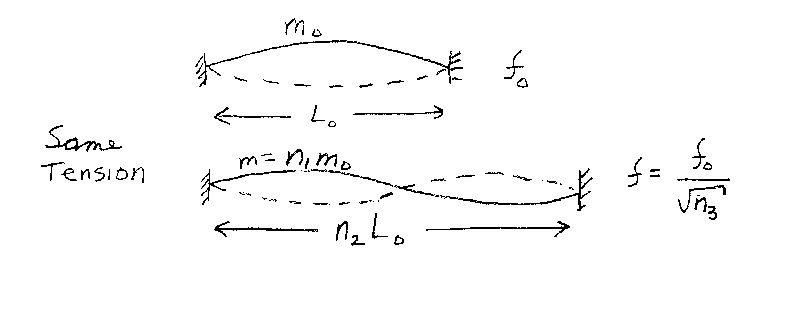Problem C15: Consider the two vibrating strings shown below. They each have the same tension T. One has a length L0 and a mass m0. This string is vibrating in its fundamental mode with a frequency f0. The second string has a mass of m=n1m0 and a length of L=n2L0. This string is vibrating in its first harmonic, as shown, with a frequency of f=f0/ √n3. what is n3? Note that n1, n2 and n3 are unitless.n1 = n2 = Input n3:

If you are currently in my class, you can record your grade by entering your name and student ID number (without the leading zeros) below and clicking on "record grade".
 First Name = Last Name = ID = Problem: